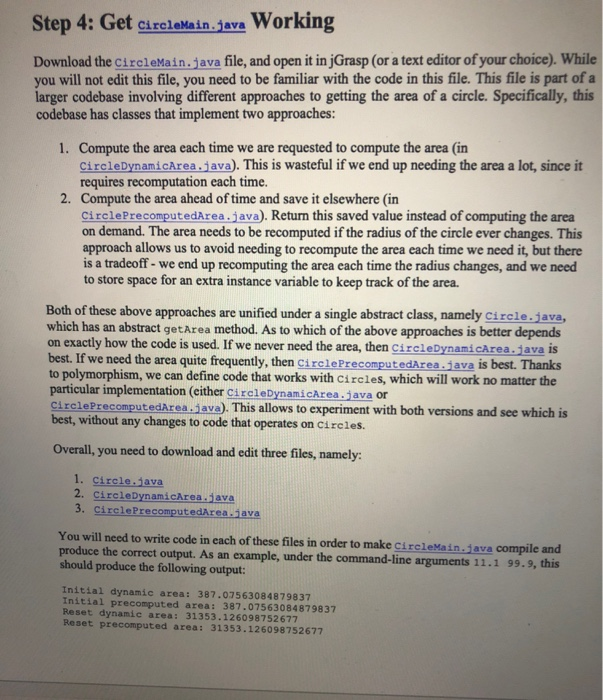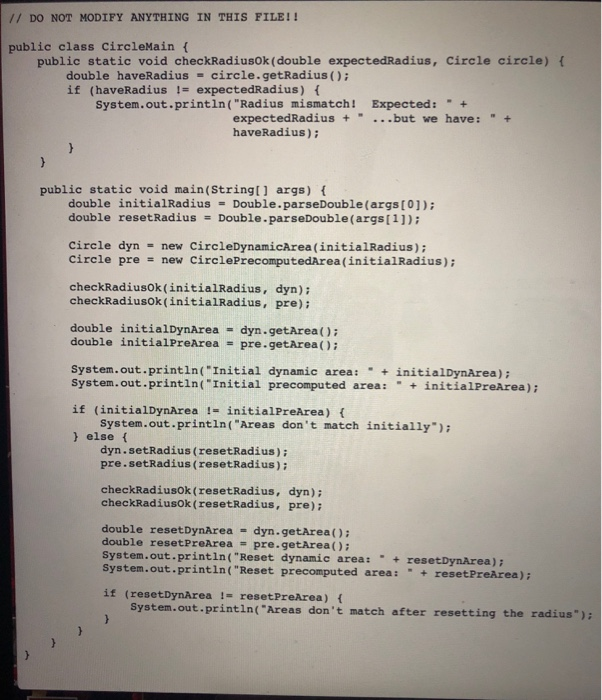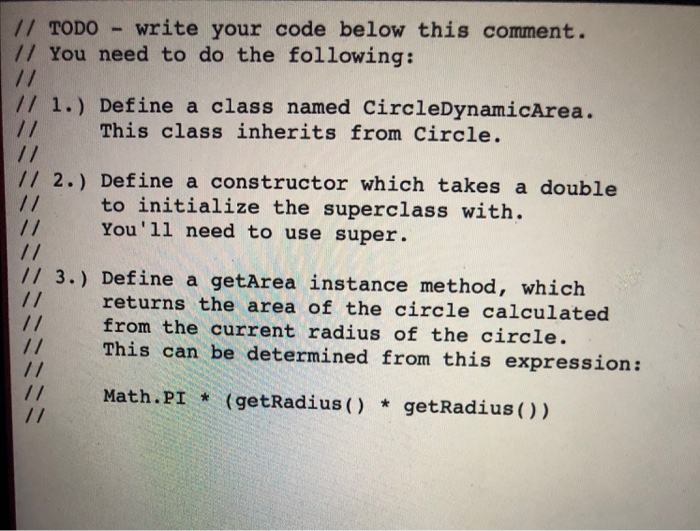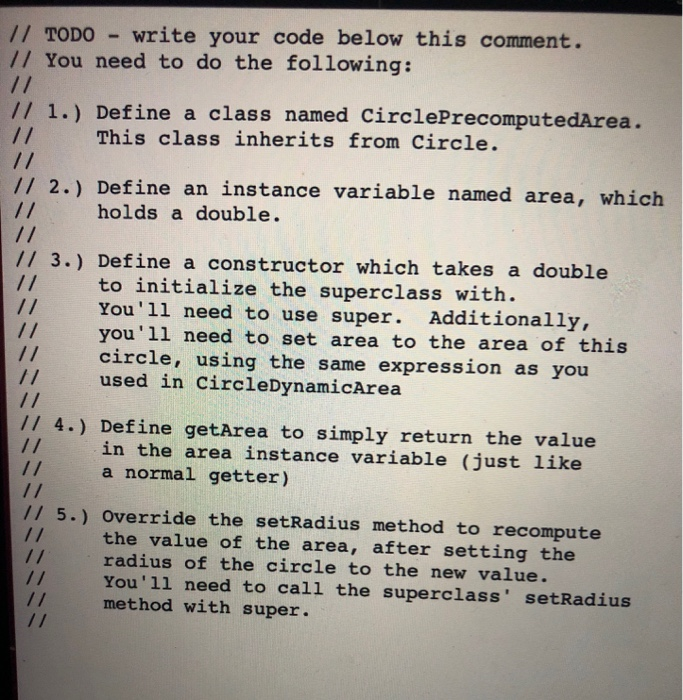# (Solved) : Step 4 Get Circlemainjava Working Download Circlemainjava File Open Jgrasp Text Editor Cho Q42763209 . . .Step 4: Get CircleMain.java Working Download the circleMain.java file, and open it in jGrasp (or a text editor of your choice). While you will not edit this file, you need to be familiar with the code in this file. This file is part of a larger codebase involving different approaches to getting the area of a circle. Specifically, this codebase has classes that implement two approaches: 1. Compute the area each time we are requested to compute the area (in Circle DynamicArea.java). This is wasteful if we end up needing the area a lot, since it requires recomputation each time. 2. Compute the area ahead of time and save it elsewhere in CirclePrecomputedArea.java). Return this saved value instead of computing the area on demand. The area needs to be recomputed if the radius of the circle ever changes. This approach allows us to avoid needing to recompute the area each time we need it, but there is a tradeoff – we end up recomputing the area each time the radius changes, and we need to store space for an extra instance variable to keep track of the area. Both of these above approaches are unified under a single abstract class, namely Circle.java, which has an abstract get Area method. As to which of the above approaches is better depends on exactly how the code is used. If we never need the area, then CircleDynamicArea.Java is best. If we need the area quite frequently, then CirclePrecomputedArea.java is best. Thanks to polymorphism, we can define code that works with Circles, which will work no matter the particular implementation (either CircleDynamicArea.java or Circle PrecomputedAreajava). This allows to experiment with both versions and see which is best, without any changes to code that operates on Circles. Overall, you need to download and edit three files, namely: 1. Circle.java 2. Circle DynamicArea.java 3. Circle PrecomputedArea.java You will need to write code in each of these files in order to make CircleMain.java compile and produce the correct output. As an example, under the command-line arguments 11.1 99.9, this should produce the following output: Initial dynamic area: 387.07563084879837 Initial precomputed area: 387.07563084879837 Reset dynamic area: 31353.126098752677 Reset precomputed area: 31353.126098752677 77 DO NOT MODIFY ANYTHING IN THIS FILE!! public class CircleMain { public static void checkRadiusok (double expectedRadius, Circle circle) { double haveRadius = circle.getRadius(); if (haveRadius != expectedRadius) { System.out.println(“Radius mismatch! Expected: ” + expectedRadius + “…but we have: ” + haveRadius); public static void main(String[] args) { double initialRadius – Double. parseDouble(args); double reset Radius = Double.parseDouble(args); Circle dyn = new CircleDynamicArea (initialRadius); Circle pre new CirclePrecomputedArea(initialRadius); checkRadiusok (initialRadius, dyn); checkRadiusok (initialRadius, pre); double initialDynArea = dyn.getArea(); double initialPreArea = pre.getArea(); System.out.println(“Initial dynamic area: ” + initialDynArea); System.out.println(“Initial precomputed area: ” + initialPreArea); if (initialDynArea ! – initialPreArea) System.out.println(“Areas don’t match initially”); } else { dyn.setRadius (resetRadius); pre.setRadius (reset Radius); checkRadiusok (resetRadius, dyn); checkRadiusok (resetRadius, pre); double reset DynArea = dyn.getArea(); double reset PreArea = pre.getArea(); System.out.println(“Reset dynamic area: ” + resetDynArea); System.out.println(“Reset precomputed area: ” + reset PreArea) if (reset DynArea – reset PreArea) { System.out.println(“Areas don’t match after resetting the radius) // TODO – write your code below this comment. 77 You need to do the following: // // 1.) Define a class named CircleDynamicArea. This class inherits from Circle. 1/ 2.) Define a constructor which takes a double 17 to initialize the superclass with. You’ll need to use super. // 3.) Define a getArea instance method, which returns the area of the circle calculated from the current radius of the circle. This can be determined from this expression: Math.PI * (getRadius() * getRadius()) // TODO – write your code below this comment. // You need to do the following: 7/ 1.) Define a class named CirclePrecomputedArea. 71 This class inherits from Circle. // 2.) Define an instance variable named area, which holds a double. 11 3.) Define a constructor which takes a double to initialize the superclass with. You’ll need to use super. Additionally, you’ll need to set area to the area of this circle, using the same expression as you used in CircleDynamicArea 17 4.) Define getArea to simply return the value in the area instance variable (just like a normal getter) 1/ 5.) Override the setRadius method to recompute the value of the area, after setting the radius of the circle to the new value. You’ll need to call the superclass’ setRadius method with super. Show transcribed image text Step 4: Get CircleMain.java Working Download the circleMain.java file, and open it in jGrasp (or a text editor of your choice). While you will not edit this file, you need to be familiar with the code in this file. This file is part of a larger codebase involving different approaches to getting the area of a circle. Specifically, this codebase has classes that implement two approaches: 1. Compute the area each time we are requested to compute the area (in Circle DynamicArea.java). This is wasteful if we end up needing the area a lot, since it requires recomputation each time. 2. Compute the area ahead of time and save it elsewhere in CirclePrecomputedArea.java). Return this saved value instead of computing the area on demand. The area needs to be recomputed if the radius of the circle ever changes. This approach allows us to avoid needing to recompute the area each time we need it, but there is a tradeoff – we end up recomputing the area each time the radius changes, and we need to store space for an extra instance variable to keep track of the area. Both of these above approaches are unified under a single abstract class, namely Circle.java, which has an abstract get Area method. As to which of the above approaches is better depends on exactly how the code is used. If we never need the area, then CircleDynamicArea.Java is best. If we need the area quite frequently, then CirclePrecomputedArea.java is best. Thanks to polymorphism, we can define code that works with Circles, which will work no matter the particular implementation (either CircleDynamicArea.java or Circle PrecomputedAreajava). This allows to experiment with both versions and see which is best, without any changes to code that operates on Circles. Overall, you need to download and edit three files, namely: 1. Circle.java 2. Circle DynamicArea.java 3. Circle PrecomputedArea.java You will need to write code in each of these files in order to make CircleMain.java compile and produce the correct output. As an example, under the command-line arguments 11.1 99.9, this should produce the following output: Initial dynamic area: 387.07563084879837 Initial precomputed area: 387.07563084879837 Reset dynamic area: 31353.126098752677 Reset precomputed area: 31353.126098752677
// TODO – write your code below this comment. 77 You need to do the following: // // 1.) Define a class named CircleDynamicArea. This class inherits from Circle. 1/ 2.) Define a constructor which takes a double 17 to initialize the superclass with. You’ll need to use super. // 3.) Define a getArea instance method, which returns the area of the circle calculated from the current radius of the circle. This can be determined from this expression: Math.PI * (getRadius() * getRadius())
// TODO – write your code below this comment. // You need to do the following: 7/ 1.) Define a class named CirclePrecomputedArea. 71 This class inherits from Circle. // 2.) Define an instance variable named area, which holds a double. 11 3.) Define a constructor which takes a double to initialize the superclass with. You’ll need to use super. Additionally, you’ll need to set area to the area of this circle, using the same expression as you used in CircleDynamicArea 17 4.) Define getArea to simply return the value in the area instance variable (just like a normal getter) 1/ 5.) Override the setRadius method to recompute the value of the area, after setting the radius of the circle to the new value. You’ll need to call the superclass’ setRadius method with super.

Answer to Step 4: Get CircleMain.java Working Download the circleMain.java file, and open it in jGrasp (or a text editor of your c…

We are the best freelance writing portal. Looking for online writing, editing or proofreading jobs? We have plenty of writing assignments to handle.